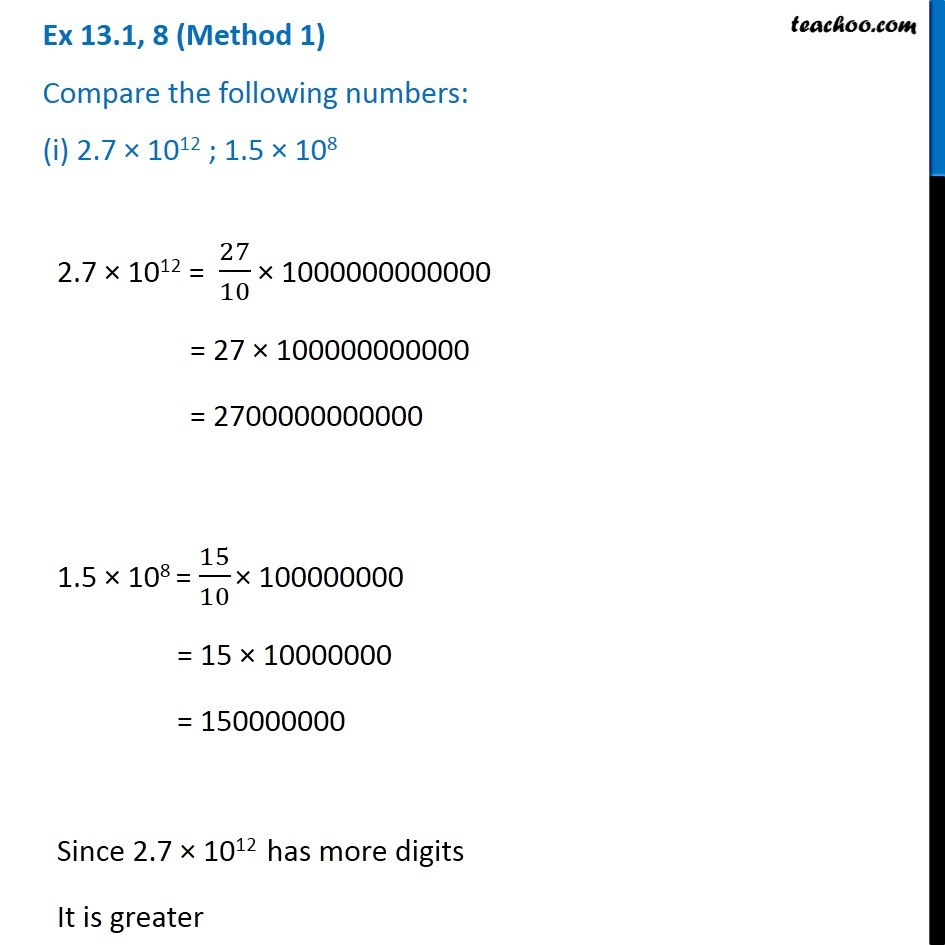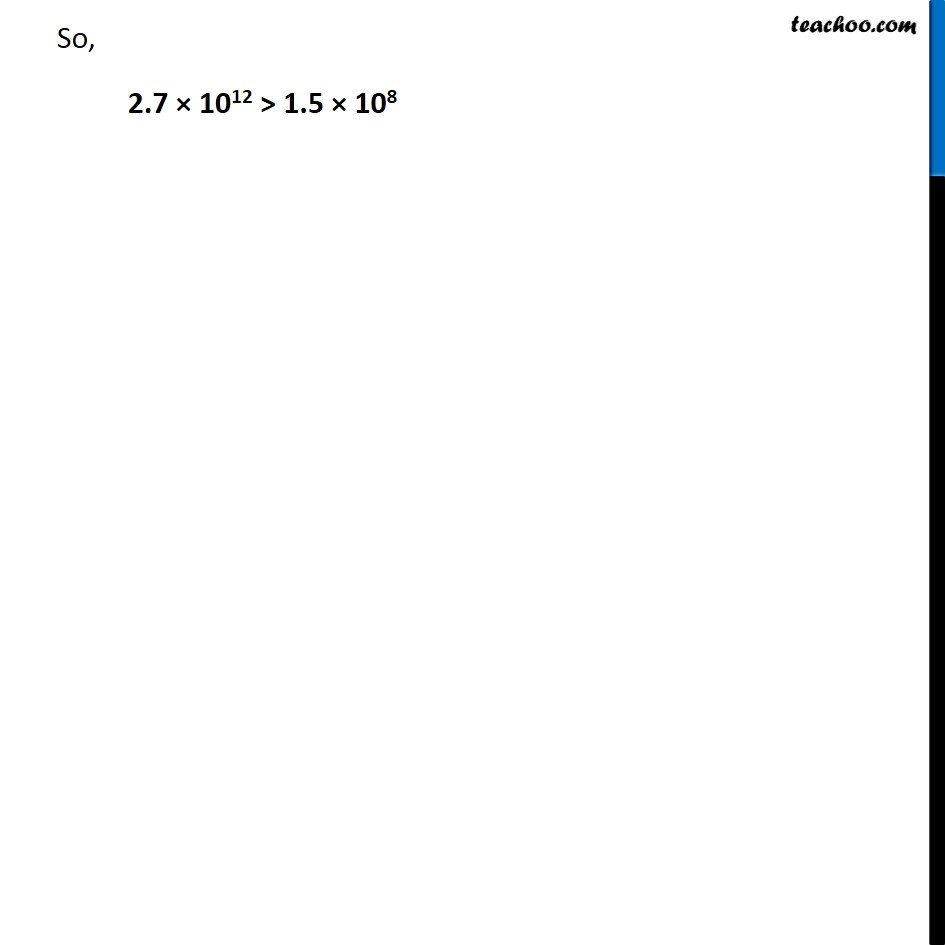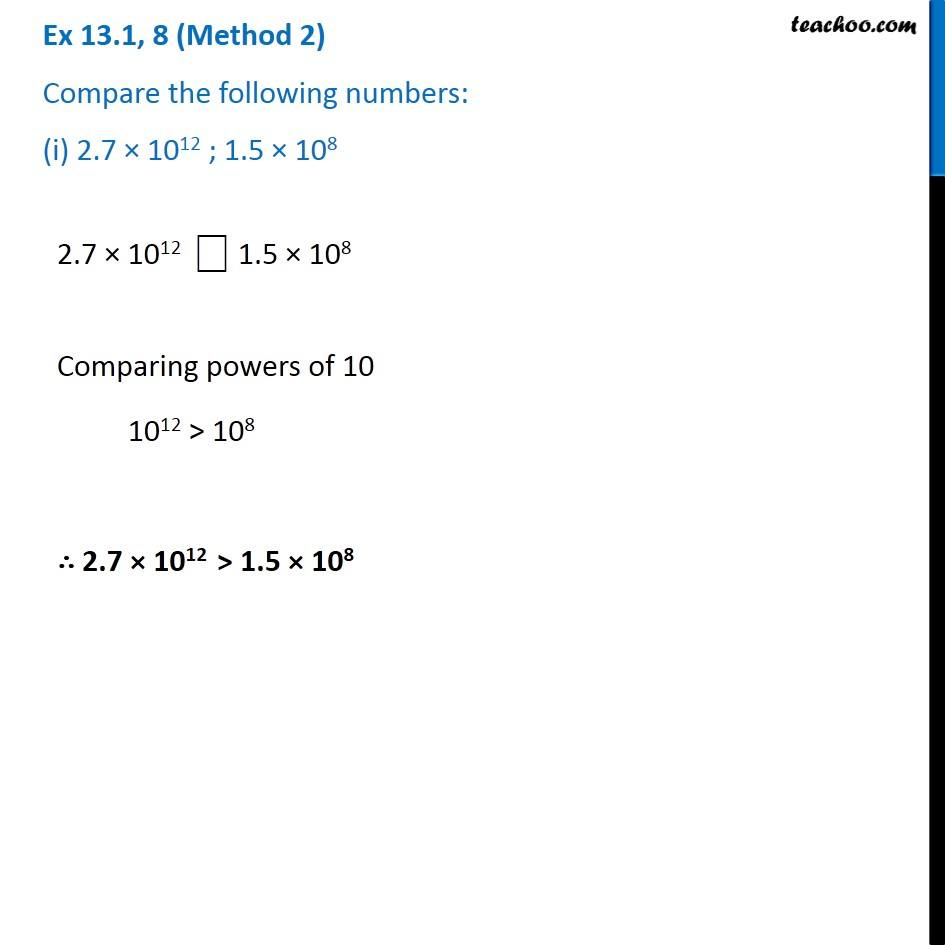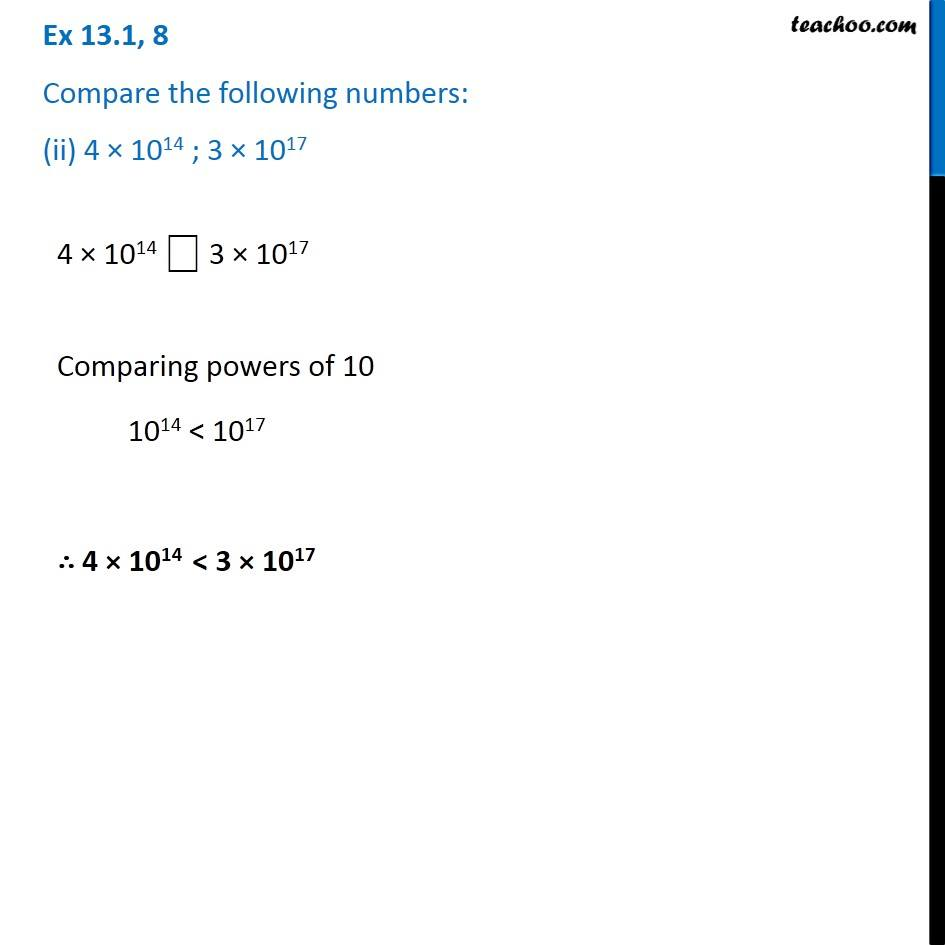1. Chapter 13 Class 7 Exponents and Powers
2. Concept wise
3. Finding which is greater

Transcript

Ex 13.1, 8 (Method 1) Compare the following numbers: (i) 2.7 × 1012 ; 1.5 × 108 2.7 × 1012 = 27/10 × 1000000000000 = 27 × 100000000000 = 2700000000000 1.5 × 108 = 15/10 × 100000000 = 15 × 10000000 = 150000000 Since 2.7 × 1012 has more digits It is greater So, 2.7 × 1012 > 1.5 × 108 Ex 13.1, 8 (Method 2) Compare the following numbers: (i) 2.7 × 1012 ; 1.5 × 108 2.7 × 1012 ⎕ 1.5 × 108 Comparing powers of 10 1012 > 108 ∴ 2.7 × 1012 > 1.5 × 108 Ex 13.1, 8 Compare the following numbers: (ii) 4 × 1014 ; 3 × 1017 4 × 1014 ⎕ 3 × 1017 Comparing powers of 10 1014 < 1017 ∴ 4 × 1014 < 3 × 1017

Finding which is greater